# Find the Smallest n Values in Excel & Google Sheets

This tutorial will demonstrate how to find the smallest n values using the SMALL function in Excel and Google Sheets.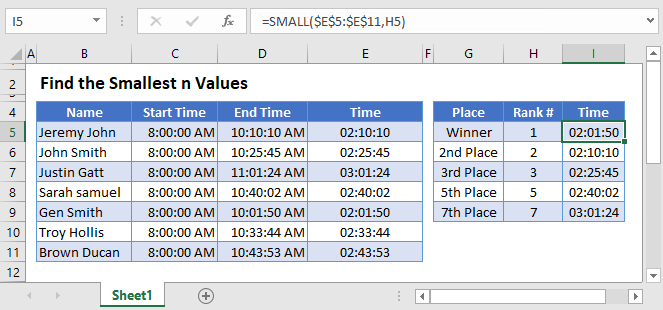## Smallest nth Value using SMALL Function

To retrieve the nth smallest numeric value from a given range (for e.g. 1st smallest, 2nd smallest etc.) use the SMALL function. Its syntax is: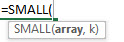This example will illustrate the process for 1st and 4th smallest value from a range.

``=SMALL(C3:C7,1)``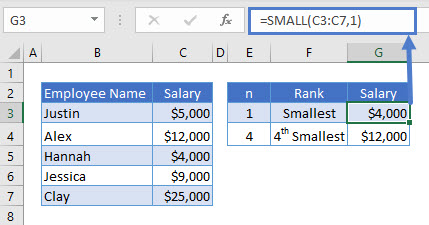The k value in the formula is modified for each nth position. For ease of understanding k is referred as ‘n’.

## SMALL Function using Cell Reference

In the previous example, the formula for each respective nth value was modified, a quicker way is to use a mixed cell reference and drag it down the table.

``=SMALL(C\$3:C\$7,\$E3)``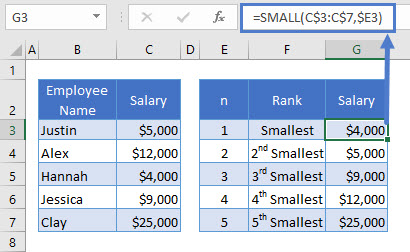Note: If the array is empty or nth position is greater than the number of records in the array, the SMALL function will return #NUM! error value.

## SMALL Function if N > Number of Records

Excel returns a #NUM! error if the nth position is greater than the number of records. Following example demonstrates the same.

``=SMALL(C3:C8,6)``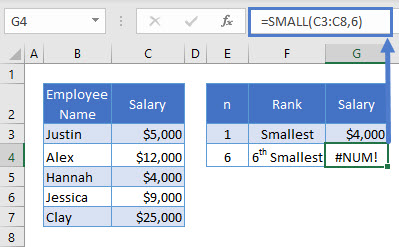## Google Sheets –Find the Smallest n Values

All of the above examples work exactly the same in Google Sheets as in Excel.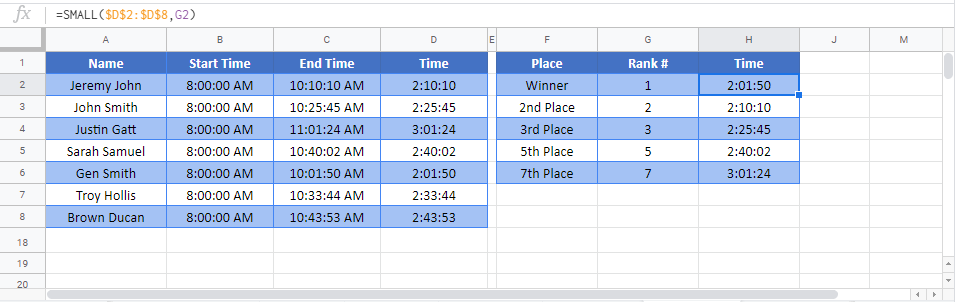### Excel Practice Worksheet

Practice Excel functions and formulas with our 100% free practice worksheets!